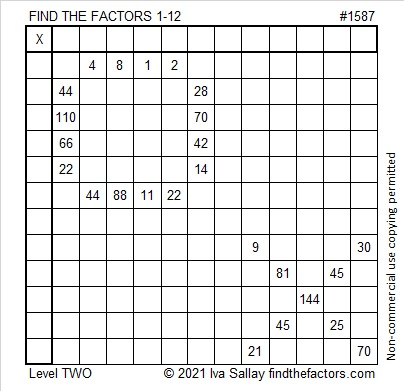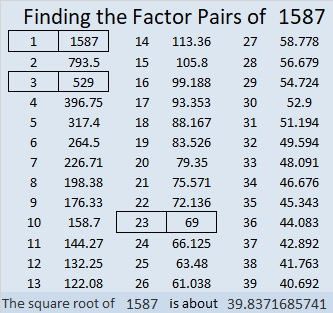# 1587 XOXOXO Hugs and Kisses OXOXOX

Contents

### Today’s Puzzle:

Hugs are often abbreviated as “O” in letters and notes, and kisses are abbreviated as “X”. This puzzle can be a Valentine’s card and will send hugs and kisses to whomever you give it.

Can you write the numbers from 1 to 12 in both the first column and the top row so that those numbers and the given clues function like a multiplication table?### Factors of 1587:

1587 is divisible by 3 because 1 + 5 + 8 + 7 = 21, a number divisible by 3.
1587 ÷ 3 = 529. Perhaps you have memorized some perfect squares and remember that 23² = 529. (If your parents invest in a 529 college plan for your education, you are more likely to have a college degree by the time you are 23. That’s how I remember it!)

• 1587 is a composite number.
• Prime factorization: 1587 = 3 × 23 × 23, which can be written 1587 = 3 × 23².
• 1587 has at least one exponent greater than 1 in its prime factorization so √1587 can be simplified. Taking the factor pair from the factor pair table below with the largest square number factor, we get √1587 = (√529)(√3) = 23√3.
• The exponents in the prime factorization are 1 and 2. Adding one to each exponent and multiplying we get (1 + 1)(2 + 1) = 2 × 3 = 6. Therefore 1587 has exactly 6 factors.
• The factors of 1587 are outlined with their factor pair partners in the graphic below.### More about the Number 1587:

1587 is the difference of two squares three different ways:
794² – 793² = 1587,
266² – 263² = 1587, and
46² – 23² = 1587.

This site uses Akismet to reduce spam. Learn how your comment data is processed.